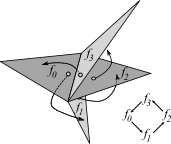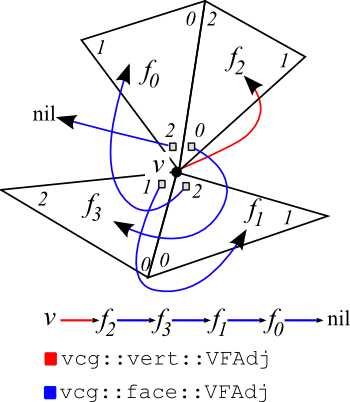\$darkmodeVCG LibraryVCG Lib does not have a unique, hard-coded, way to encode the adjacencies among triangles and edges. It all depends on which attributes are stored with the simplices and how they are used. In the previous examples the definition of face has always included the attribute vcg::face::VertexRef, which stores 3 pointers to MyVertex accessible with the member function V() (implementing a plain Indexed Data Structure). Almost all of the algorithms currently implemented in vcglib assume that vcg::face::VertexRef is present. So, if your type MyFace does not include the attribute vcg::face::VertexRef, the definition will be correct, but almost no algorithm will work.

There are other adjacency relations that can be useful to navigate a mesh, for example to collect the one-ring neighborhood of a vertex. vcgib has two main adjacency relations face-face and vertex-face which are explained in the following, along with the attributes they use.

The face-to-face adjacency, stored in the attribute for the faces vcg::face::FFAdj , encodes the adjacency between faces through edges. The image below shows two triangle faces with the convention adopted for indexing vertexes and edges.

The vertexes are numbered from 0 to 2 in CCW direction and the edge i = 0..2 is the edge whose extremes are i and (i+1) mod 3. Therefor, in the below example, the common edge between faces f0 and f1 is the edge 1 of the face f1 and the edge 0 of the face f0.

vcg::face::FFAdj stores, for the i-th edge of the face f:

• `FFp(i)`: a pointer to the face sharing the i-th edge of f. If that edge is a border than points to the face f itself
• `FFi(i)`: the index of edge that corresponds to i-th edge of f in the pointed faceIndexing of vertexes and edges

So for the above example, assuming that `f0` and `f1` are the two pointers to faces, the following relations hold:

```f1->FFp(1) == f0
f1->FFi(1) == 0

f0->FFp(0) == f1
f0->FFi(0) == 1
```

Note that to be precise we should say that `FFp(i)` points to a adjacent face, not to the adjacent face. The reason is that, in case of non-manifoldness, there could be more than two faces on the same edge, and this is seamlessly supported by VCG Lib. In the picture below is shown an example with 4 faces incident to the same edge.Non manifold edge

In this case the FF adjacencies are arranged to form a circular list (not necessarily sorted with the angle around the edge). This is done by the VCG Lib function that update these values ( vcg::tri::UpdateTopology::FaceFace (MeshType & m)). In this way VCG Lib provides a way to check if a mesh is manifold on a specific edge because the FF adjacency relation is mutual, i.e. the face f0 points to the face f1 which points to the face f0, if and only if the mesh is manifold over the corresponding edge.

```bool IsManifold(MyFace *f,int e) { return (f0 == f0->FFp(0)->FFp(f0->FFi(0)))}
```

## Pos

The Pos is the VCG Lib implementation of the Cell-Tuple{ref}. Here we give a as short as possible definition, trading formalisms for space. A Pos in a triangle mesh is a triple made of a vertex: pos = (v,e,f) , such that v is an extreme of e and e belong to the face f . The figure shows few pos in a triangle mesh as small triangles "pointing" to a vertex, "leaning" against an edge and inside a face. For example c0=(v,e0,f).

A very nice property is that given a pos c, there is only another neighbor pos c' that can be obtained from c changing only one of the elements of the triple.

We call the operation of passing from a pos to one of its neighbors Flip and write FlipV, FlipE and FlipF to indicate that the flipped element is the vertex, the edge or the face respectively.

For example consider c1: there is only another pos which is the same as c0 except for the vertex component of the triple, and it is c2. For brevity, we write c2 = FlipV(c1).

In the left of the table some other examples are shown just to make this point clear.Pos Example
```c2 = FlipV(c1)
c0 = FlipE(c1)
c3 = FlipF(c0)

CCW around v
c4 = FlipE(FlipF(c0))
c5 = FlipE(FlipF(c4))
Bounce
c6 = FlipE(FlipF(c5))
CW around v
c3 = FlipE(FlipF(c6))
c1 = FlipE(FlipF(c3))
Bounce
c0 = FlipE(FlipF(c1))
```

Note that concatenating two flips: FlipF and FlipE we obtain a transition from a pos to the next in counterclockwise or in clockwise sense, depending if the starting pos is on the CCW edge of the face with respect to the vertex or not. Also note that, thanks to how FF adjacency is defined, when a pos is on the border, it bounces back. This pair of flip are widely used in the VCG Lib to run over the one ring neighborhood of manifold vertices.

The following code snippet shows how to use the pos to iterate around a vertex:

```sf/apps/sample/trimesh_pos_demo/trimesh_pos_demo.cpp

#include <vcg/simplex/face/pos.h> // include the definition of pos

...includes to define your mesh type

class MyVertex: ...
class MyFace: public vcg::FaceSimp2<MyVertex,MyEdge,MyFace, vcg::face::VertexRef, vcg::face::FFAdj>{};

void OneRingNeighborhood( MyFace * f)
{
MyVertex * v = f->V(0);
MyFace* start = f;
vcg::face::Pos<MyFace> p(f,0,v);// constructor that takes face, edge and vertex
do
{
p.FlipF();
p.FlipE();
}while(p.f!=start);
}
```

Two important notes:

We arbitrarily picked f->V(0) as pivot vertex. In general one may want to start knowing the vertex. This is done by including the attribute vcg::vertex::VFAdj which basically associates to each vertex pointer to one of the faces incident on it. See the VF Adjacency for details. This implementation does not work if the vertex is on the border. Just try with the example: from the pos c4 it would find c5,c6,c3 which is in the same face as c4. Of course this does not happen if you use the pos itself as a guard and not just the face. However, even in this case, you would obtain the sequence of pos: c5,c6,c3,c1,c0,c4 corresponding to the faces f2,f2,f1,f0,f0,f1 which probably is not what you want. VCG Lib provides a variation of pos that solves this problem

## Jumping Pos

The Jumping Pos works exactly like the Pos, only it does not bounce when it encounters the border. Instead, it jump around the vertex as if the to border faces sharing the vertex (faces f0 and f2 in the image) were adjacent.

sf/apps/sample/trimesh_pos_demo/trimesh_pos_demo.cpp
#include <vcg/simplex/face/jumping_pos.h> // include the definition of jumping pos
//...includes to define your mesh type
//class MyVertex: ...
void OneRingNeighborhoodJP( MyFace * f)
{
MyVertex * v = f->V(0);
MyFace* start = f;
vcg::face::JumpingPos<MyFace> p(f,0,v);// constructor that takes face, edge and vertex
do
{
p.NextFE();
}while(p.f!=start);
}

VCG Lib implements vertex-to-face adjacency, i.e. given a vertex v we can retrieve all the faces incident to v. Let v_star =(f0,f1,f2,...,fk) be the set faces incident to v arranged in a sequence (with no preferred criteria). VCG Lib allows to retrieve v_star in optimal time ( O( star_v) ) by using the following attributes:

• vcg::vertex::VFAdj which is a vertex attribute containing a pointer to f0
• vcg::face::VFAdj which is a face attribute containing a pointer to the next face in the list v_star for each of its 3 vertices

These two attributes are not only a pointers, they also contain an index referring the index of the vertex in the pointed face in the same style as the vcg::face::FFAdj does. The picture below shows a practical example: Similarly to the Face-Face adjacency you need to initialize it using vcg::tri::UpdateTopology::VertexFace(MeshType & m).```v.VFp() == f2
v.VFi() == 0

f2->VFp(0) == f3
f2->VFi(0) == 1
f3->VFp(1) == f1
f3->VFi(1) == 2
f1->VFp(2) == f0
f1->VFi(2) == 2
f0->VFp(2) == NULL
f0->VFi(2) == -1
```

## VFIterator

VFIterator is a simple iterator to run over the faces in the one-ring neighborhood of a vertex using the VF Adjacency (it is just like Pos for the FF Adjacency) The following code snippet shows how to use the VFIterator:

sf/apps/sample/trimesh_pos_demo/trimesh_vfiter_demo.cpp
#include <vcg/simplex/face/pos.h> // include the definition of VFIterator
//...includes to define your mesh type
class MyVertex: public vcg::VertexSimp2<MyVertex,MyEdge,MyFace, vcg::vertex::VFAdj /*,... other attributes*/ >{};
void OneRingNeighborhoodVF( MyVertex * v)
{
vcg::face::VFIterator<MyFace> vfi(v); //initialize the iterator tohe first face
for(;!vfi.End();++vfi)
{
MyFace* f = vfi.F();
// ...do something with face f
}
}

## Stars and Rings

We have a few handy functions to recover the set of faces/vertices incident on vertex: